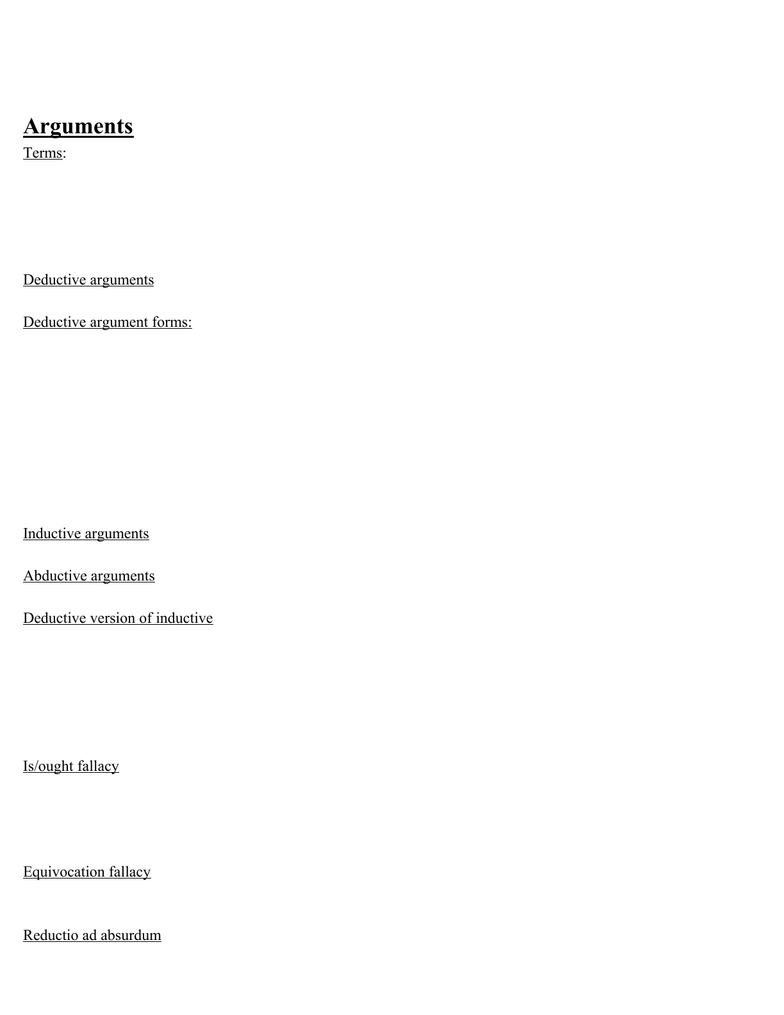Study Guides (380,000)
US (220,000)
UMD (10,000)
PHIL (70)
Final

# PHIL 140 Study Guide - Final Guide: Modus Ponens, Reductio Ad Absurdum, Modus Tollens

Department
Philosophy
Course Code
PHIL 140
Professor
Kirilov
Study Guide
Final

This preview shows page 1. to view the full 4 pages of the document.PHIL140 Final Exam Study Guide & Cheat Sheet
Contemporary Moral Issues, Spring 2019
Arguments
Terms:
Premise = indicated by phrases such as since, because, for, this follows from”
Conclusion = indicated by phrases such as therefore, so, thus
Validity = conclusion follows from premises
Soundness= valid AND has true premises
Deductive arguments = conclusion follows from premises with logical necessity, supposing the premises are true;
impossible to have false conclusion
Deductive argument forms:
- Modus Ponens: Premise 1. if A, then B → Premise 2. A → Conclusion: B
- Modus Tollens: P1. if A, then B → P2. not A → C. not B
- Disjunctive Syllogism: P1. A or B → P2. not A → C. B
- Hypothetical Syllogism: P1. if A, then B → P2. if B, then C → C. B
- Introduction to Conjunction: P1. A → P2. B → C. A and B
- Elimination of Conjunction: P1. A and B → C. B
- Introduction of Disjunction: P1. A or B → C. A
- Double Negation Elimination: P1. if it is not the case that it is not A → C. it is A
Inductive arguments = valid if conclusion follows with high probability (generalization); i.e.
Abductive arguments = valid if conclusion is the BEST explanation; simplicity, explanatory power, likelihood
Deductive version of inductive = sample population(S), overall population(O), property(P), distribution (D); similar to
Modus Ponens
- P1. D% off members of S have property P and S is s representative sample of O with respect to P.
- P2. If D% of members of S have P and S has a high chance of being a representative sample, there’s a high
chance that D% of O have property P.
- C. From P1 and P2 follows that there’s a high chance D% of O have P.
Is/ought fallacy = attempting to derive an evaluative fact from non-evaluative fact; i.e. P1. water is H2O → C. water is
good
- Evaluative - what ought to be, what’s valuable, what’s better; non-evaluative = doesn’t tell anything about
values
Equivocation fallacy - key term changes meaning in argument (i.e. feathers are light→ what’s light isn’t dark →
feathers can’t be dark); invalid
Reductio ad absurdum - reduces position being criticized to absurdity (false state); i.e. assume P is true → from this,
deduce that Q is true→ also, deduce that Q is false→ thus, P implies both Q and not Q (a contradiction, which is
necessarily false) → therefore, P itself must be false
###### You're Reading a Preview

Unlock to view full version